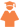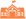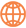# How to get Good Scores in SAT Test

In order to view the results the Pakistani students must visit the official website of College Board, enter SAT login of Pakistani students and password then view their SAT results of Pakistani students in 2020.

Official score reports are provided about five weeks after the SAT test is administered. Score is calculated in two steps:

## A raw SAT score is calculated.

First raw score is calculated by the SAT score calculator for Pakistani students which consist of the following -

One point is added for each multiple-choice question which is answered correctly. For multiple-choice questions answered incorrectly, about 1/4 point is subtracted - No points are subtracted for incorrect answers to the mathematics questions requiring student- they are known as produced responses. No points are subtracted for omitted questions.

After giving individual points, the total points answered wrong are subtracted from the number answered correctly. If the resulting score is a fraction, it is rounded to the nearest whole number—1/2 or more is rounded up; less than 1/2 is rounded down. Questions in the SAT equating section do not count toward the score.

The raw score is converted to the College Board SAT Pakistan 200- to 800-point scaled score by a statistical process known as equating.

## The SAT Score report includes:

In SAT report one point is added for each multiple-choice question which is answered correctly. A breakdown of the student's scores, including the types of questions, level of difficulty, and how many in each group of questions the student answered correctly, answered incorrectly, or omitted Information about what those scores mean, with enhanced percentile information to help better compare the student’s performance with other groups of test-takers.

After the SAT score release time in Pakistan is when official SAT score reports are provided about five weeks after the SAT test is administered. They are sent to the student's high school, if the student entered the correct high school code number when registering.

The SAT score is only a report of their preparation of SAT exam.

## A Good SAT Score –

The good SAT score range of Pakistani students varies from university to university or student to student. The top colleges require a SAT score of about 850 to 1600.

• Text
• Image
• VideoXX

X

X

### Register Type

Please Provide following information to Register

•Student
•College/University
•Consultant
X

### Ask a qusetion from a expert

Please Provide maximum information so that the best advice can be given